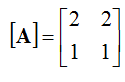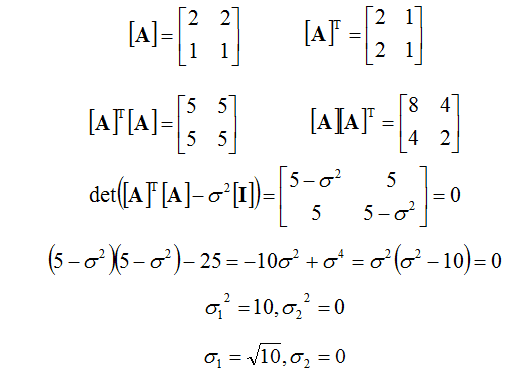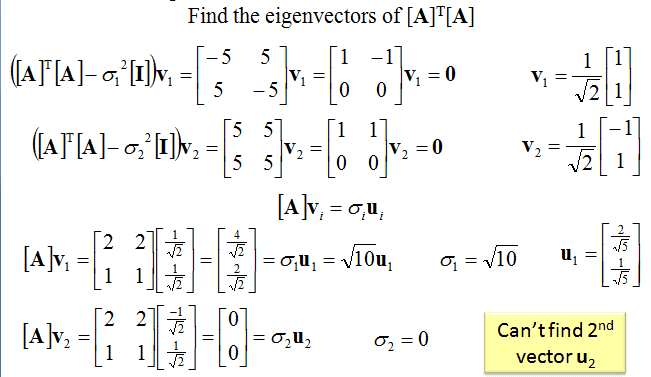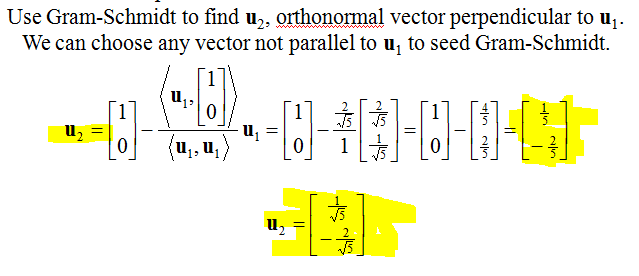# Linear Algebra - Singular Value Decomposition Problem

## Homework Statement

Find the SVD of## The Attempt at a Solution

I'm stuckMy question is why in the solution it originally finds u_2=[1/5,-2/5]' but then says u_2=[1/sqrt(5),-2/sqrt(5)]'. I don't see what math was done in the solution to change the denominator from 5 to square root 5.

General Question - When finding the singular value...
(sigma_1)^2 = constant, why do we only consider the positive root
sigma_1 = sqrt(constant)
because the solution to the problem is
sigma_1 = +/- sqrt(constant)

vela
Staff Emeritus
Homework Helper
My question is why in the solution it originally finds u_2=[1/5,-2/5]' but then says u_2=[1/sqrt(5),-2/sqrt(5)]'. I don't see what math was done in the solution to change the denominator from 5 to square root 5.
You're looking for an orthonormal basis.

General Question - When finding the singular value...
(sigma_1)^2 = constant, why do we only consider the positive root
sigma_1 = sqrt(constant)
because the solution to the problem is
sigma_1 = +/- sqrt(constant)

What's the definition you're using of a singular value?

Sorry I don't understand. It has to be orthornormal to u_1 so taking the dot product with u_1 and u_2 has to be zero and that's were it comes from?

I'm talking about just when it finds sigma_1 and sigma_2 why don't we consider the negative square root into our calculation when we find SVD? Like when we form the sigma matrix it's the singular values in a diagonal matrix, so I just don't understand really why we don't consider the negative root.

vela
Staff Emeritus
Homework Helper
Sorry I don't understand. It has to be orthornormal to u_1 so taking the dot product with u_1 and u_2 has to be zero and that's were it comes from?
No.

I'm talking about just when it finds sigma_1 and sigma_2 why don't we consider the negative square root into our calculation when we find SVD? Like when we form the sigma matrix it's the singular values in a diagonal matrix, so I just don't understand really why we don't consider the negative root.
Look up the definition of a singular value..

blue_leaf77